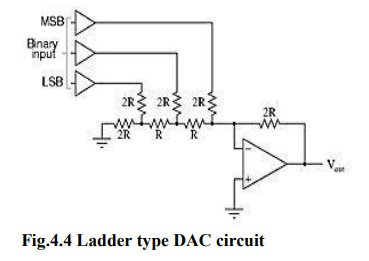Home | | Linear Integrated Circuits | R-2R Ladder DAC

## Chapter: Linear Integrated Circuits : Analog to Digital And Digital to Analog Converters

An enhancement of the binary-weighted resistor DAC is the R-2R ladder network.

An enhancement of the binary-weighted resistor DAC is the R-2R ladder network. This type of DAC utilizes Thevenin’s theorem in arriving at the desired output voltages.

A disadvantage of the former DAC design was its requirement of several different precise input resistor values: one unique value per binary input bit.

The R-2R network consists of resistors with only two values - R and 2xR. If each input is supplied either 0 volts or reference voltage, the output voltage will be an analog equivalent of the binary value of the three bits. VS2 corresponds to the most significant bit (MSB) while VS0 corresponds to the least significant bit (LSB).Vout =  - (VMSB + Vn + VLSB) = - (VRef + VRef/2 + VRef/ 4)Tags : Digital to Analog Converters , Linear Integrated Circuits : Analog to Digital And Digital to Analog Converters
Study Material, Lecturing Notes, Assignment, Reference, Wiki description explanation, brief detail
Linear Integrated Circuits : Analog to Digital And Digital to Analog Converters : R-2R Ladder DAC | Digital to Analog Converters